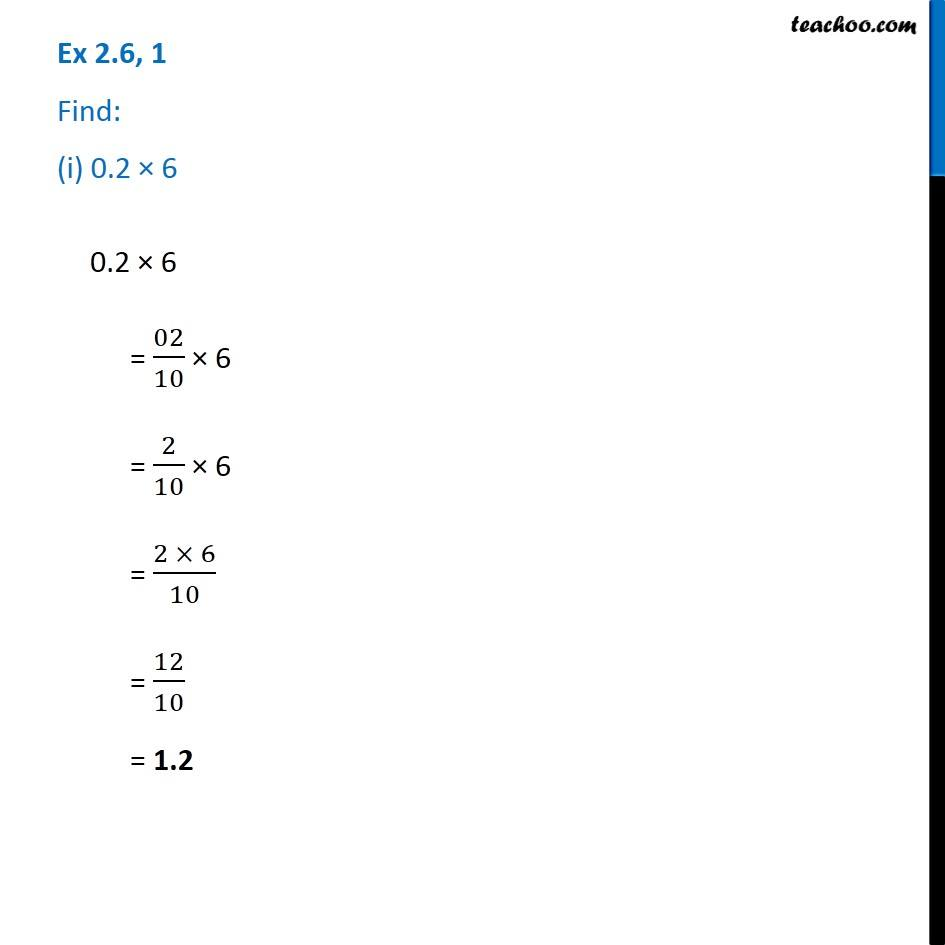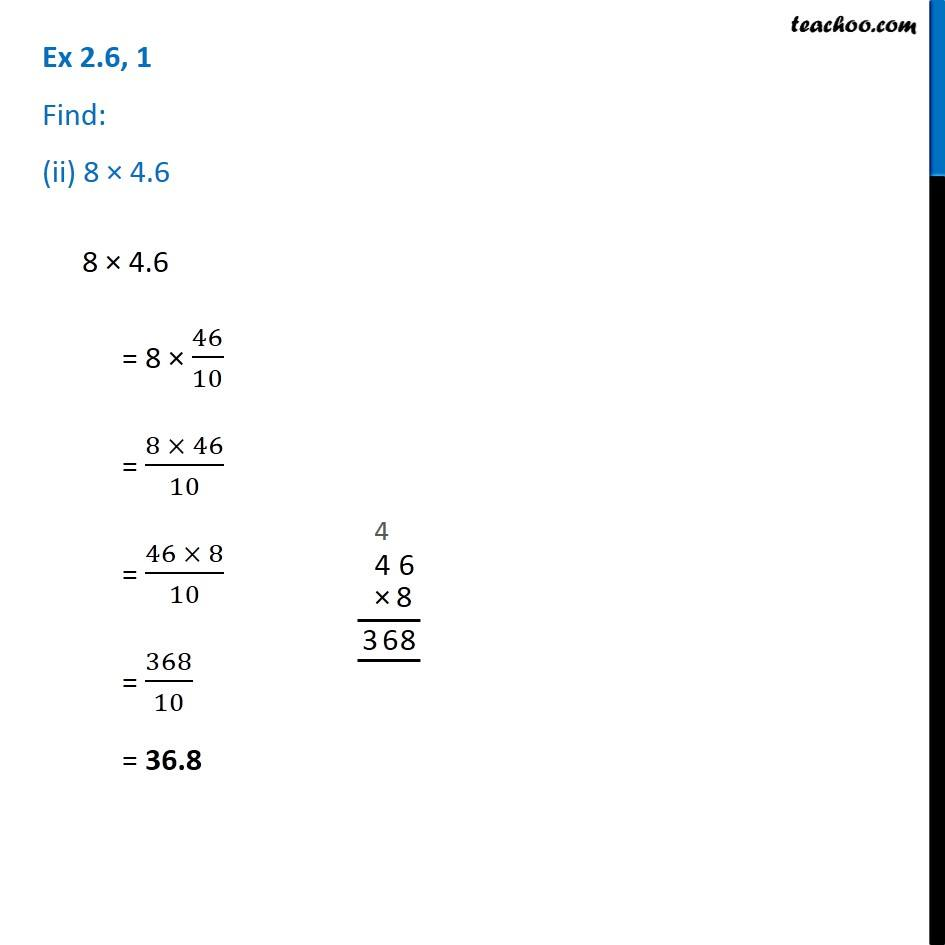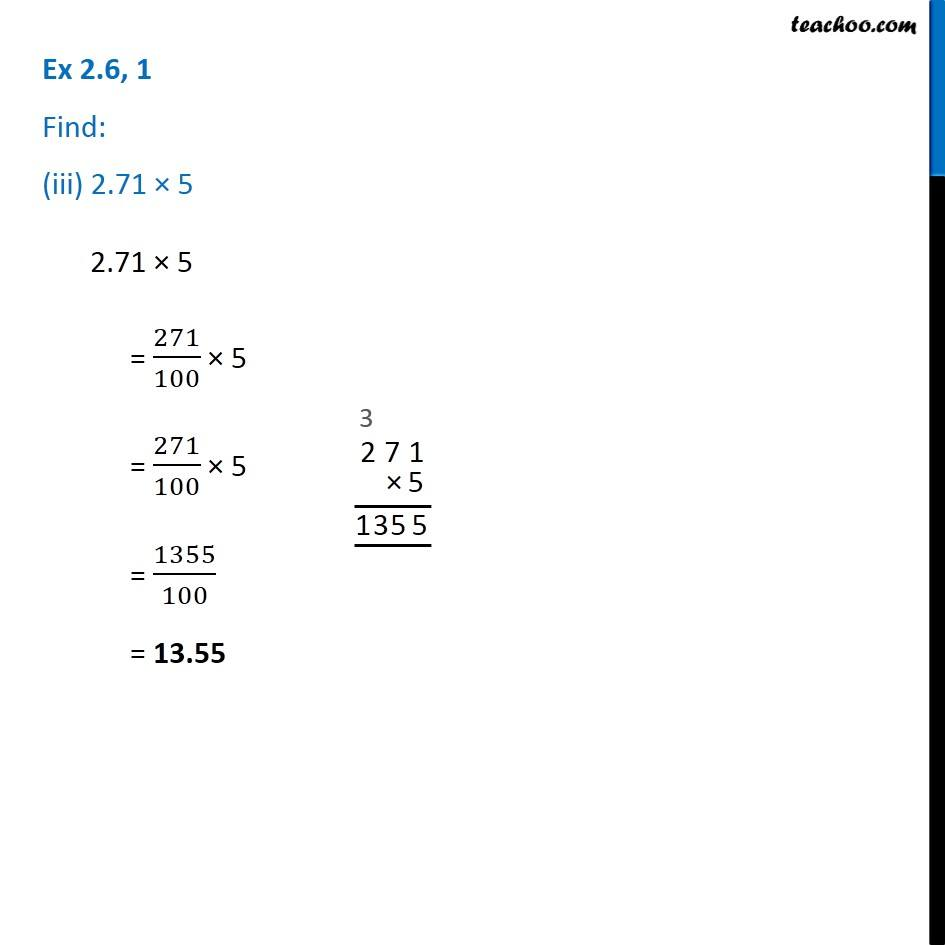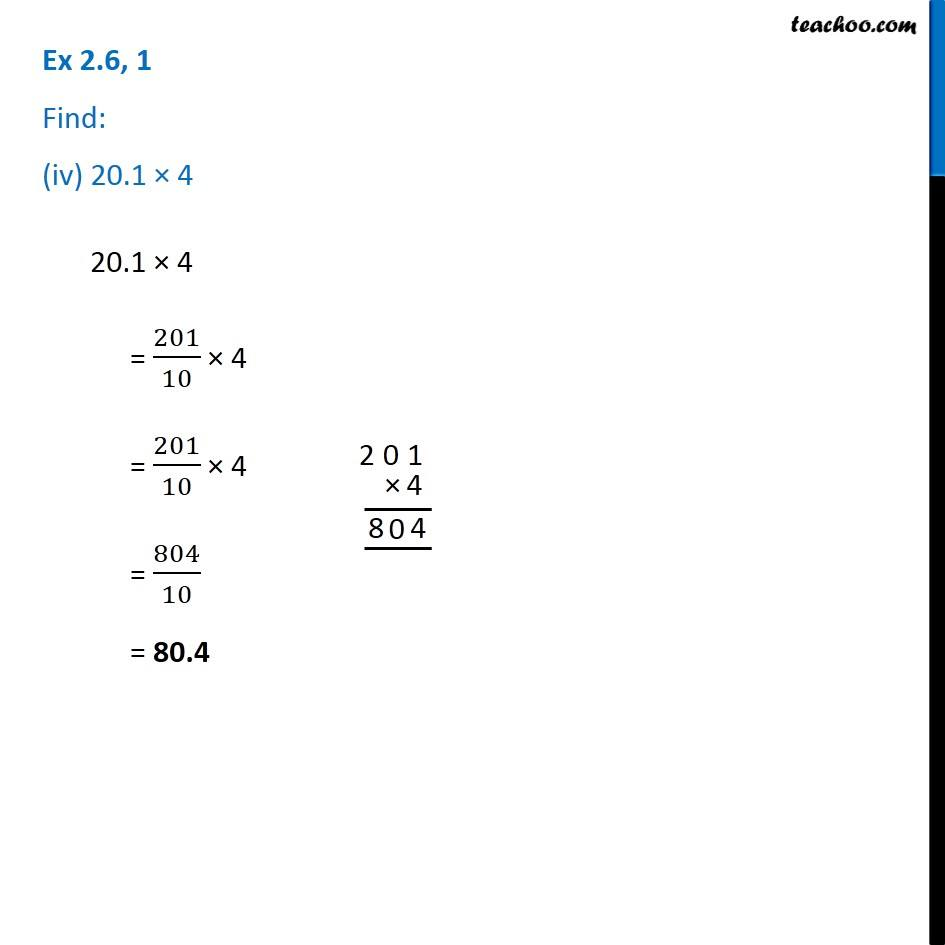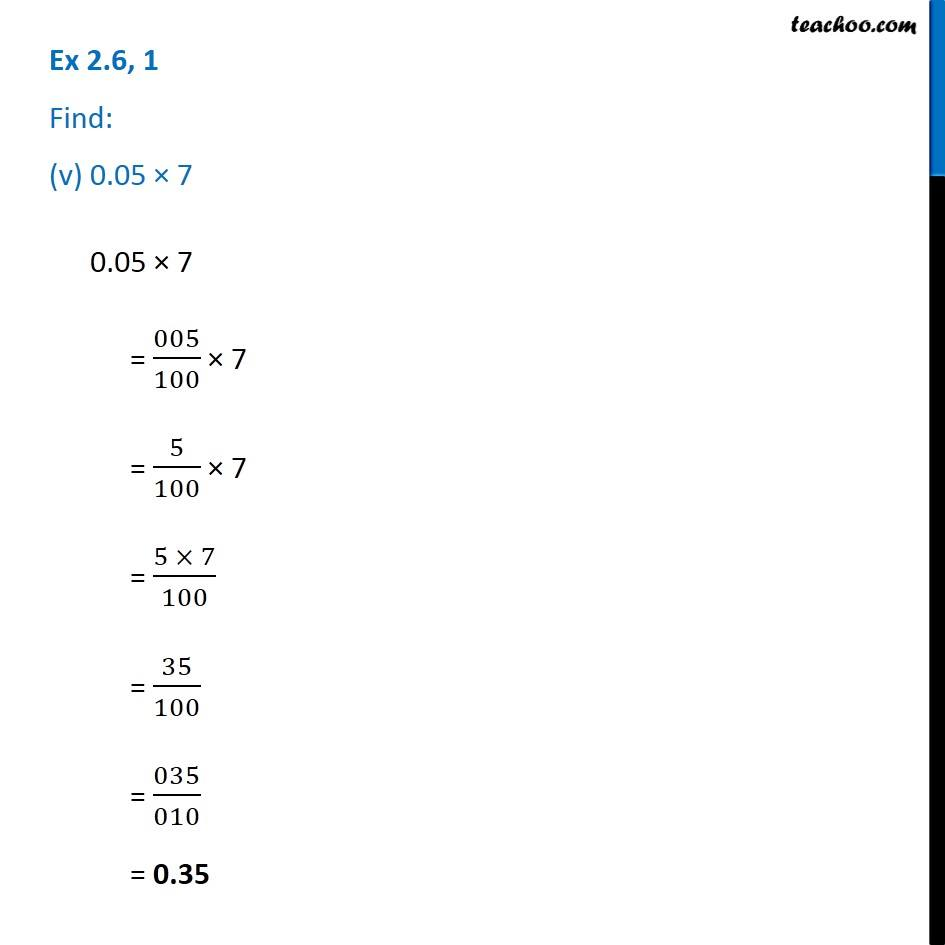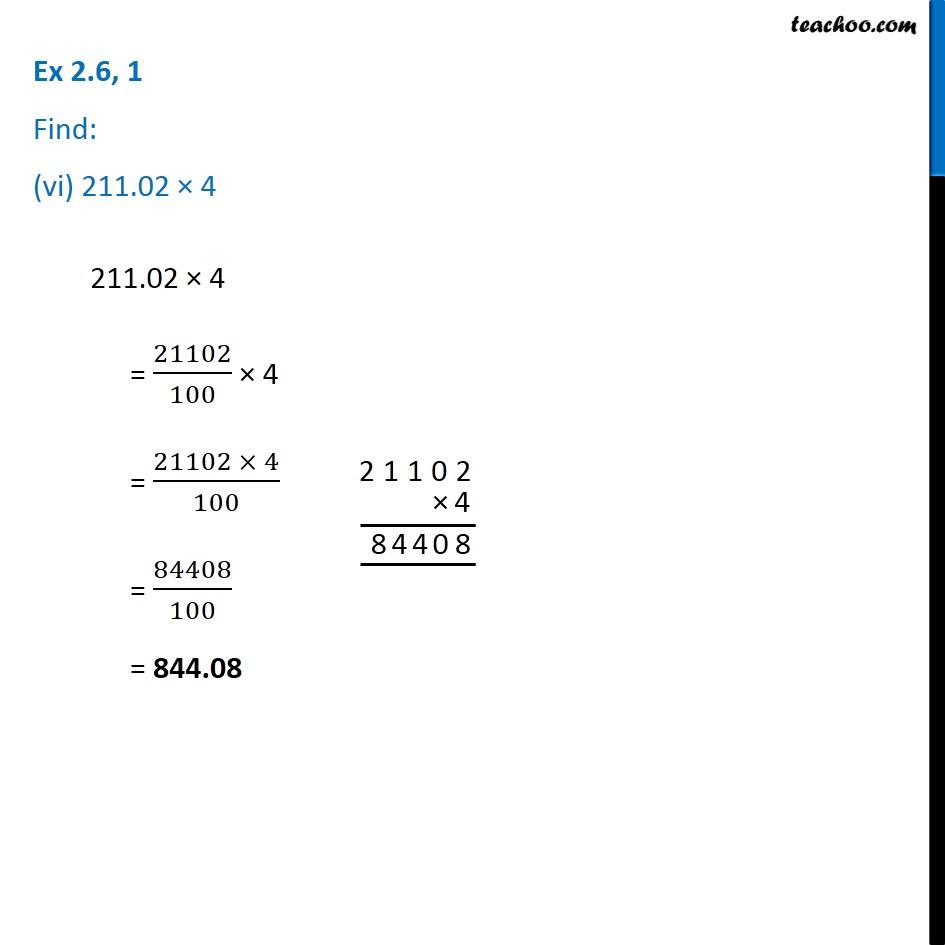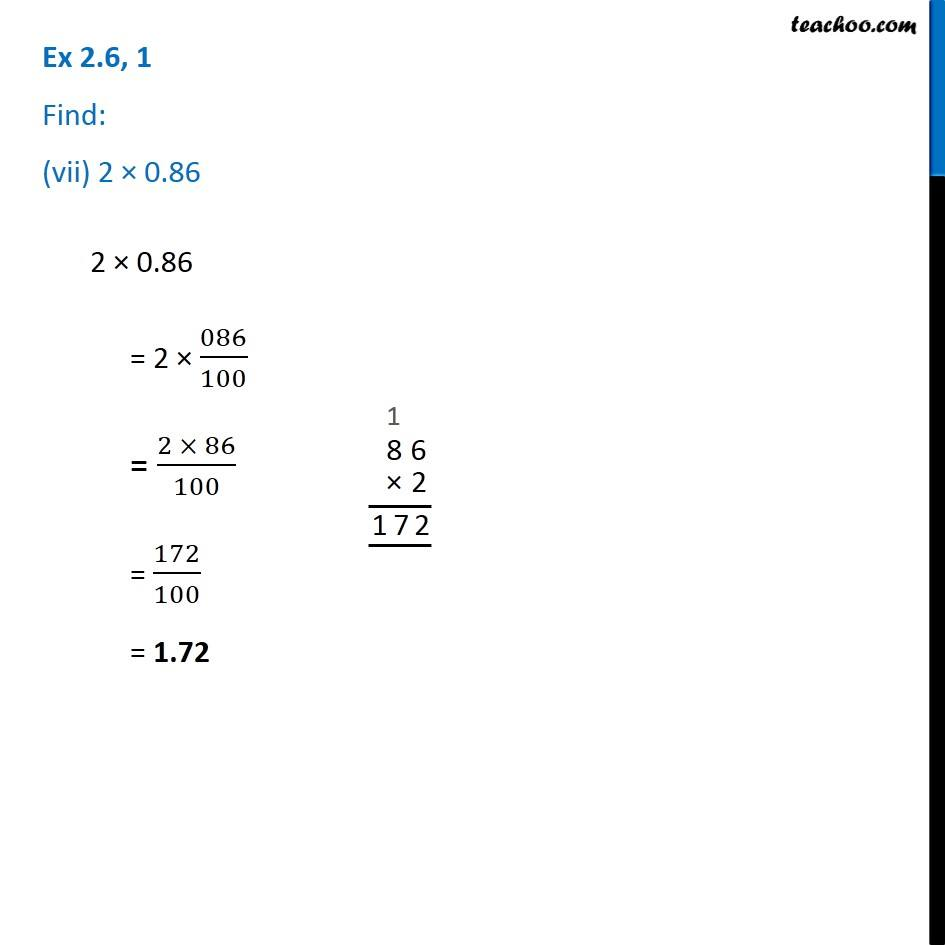1. Chapter 2 Class 7 Fractions and Decimals
2. Concept wise
3. Multiplication of Decimals

Transcript

Ex 2.6, 1 Find: (i) 0.2 × 6 0.2 × 6 = 02/10 × 6 = 2/10 × 6 = (2 × 6)/10 = 12/10 = 1.2 Ex 2.6, 1 Find: (ii) 8 × 4.6 8 × 4.6 = 8 × 46/10 = (8 × 46)/10 = (46 × 8)/10 = 368/10 = 36.8 Ex 2.6, 1 Find: (iii) 2.71 × 5 2.71 × 5 = 271/100 × 5 = 271/100 × 5 = 1355/100 = 13.55 Ex 2.6, 1 Find: (iv) 20.1 × 420.1 × 4 = 201/10 × 4 = 201/10 × 4 = 804/10 = 80.4 Ex 2.6, 1 Find: (v) 0.05 × 7 0.05 × 7 = 005/100 × 7 = 5/100 × 7 = (5 × 7)/100 = 35/100 = 035/010 = 0.35 Ex 2.6, 1 Find: (vi) 211.02 × 4 211.02 × 4 = 21102/100 × 4 = (21102 × 4)/100 = 84408/100 = 844.08 Ex 2.6, 1 Find: (vii) 2 × 0.86 2 × 0.86 = 2 × 086/100 = (2 × 86)/100 = 172/100 = 1.72

Multiplication of Decimals